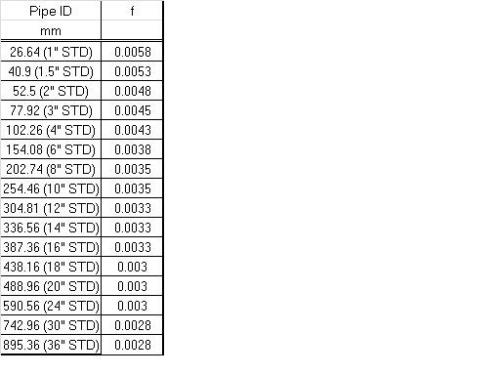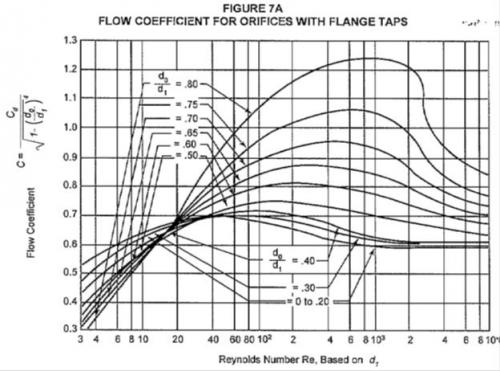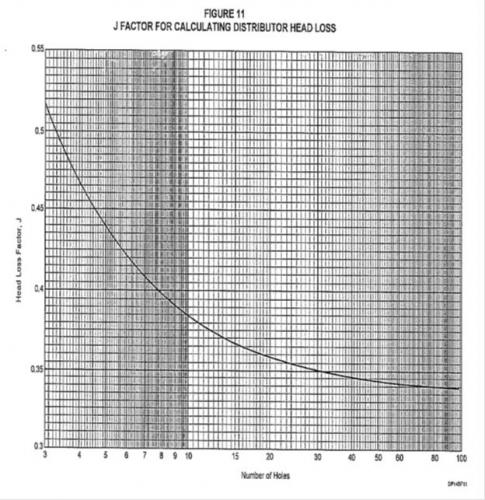6 votes

# Perforated Pipe Distributor Sizing CalculationsPerforated Pipe Distributors have been discussed many times on “Cheresources”. How to design a perforated pipe distributor also known as a sparger has been a frequent question on the forum. Some general replies without providing in-depth methodology of sizing a distributor can be seen in the queries raised.

Perry’s Handbook (8th Edition) has a brief sub-section on perforated pipe distributors in Section 6, Fluid & Particle Dynamics which I had gone through critically but which was not to my satisfaction.

Since I do have a penchant of finding and developing engineering calculations, I was continuously on the look-out for something related to perforated pipe distributors. Last week I struck gold, when I found a company design manual providing detailed calculations for perforated pipe distributors. I had a critical look at it and found it to be better than anything I had found and read earlier related to perforated pipe distributors. I could even develop an excel workbook for perforated pipe distributor from this very informative design procedure.

Today’s blog entry is meant to provide the stepwise calculations for a perforated pipe distributor including the design equations. Both liquid and gas pipe distributors are covered. Gas pipe distributors follow the same design steps as that for liquid pipe distributors except for some minor variations in the design procedure. Both Metric units and USC units have been provided in the methodology.

Let us straight away get to the steps for sizing a Perforated Pipe Liquid distributor:

Step 1:
Initially set the pipe size of the pipe distributor, same as the pipe size feeding the pipe distributor

Step 2:
Calculate the Reynolds number, Rei, of the inlet stream to the pipe distributor using the following equation:

Metric Units:
Rei = 1.27*Q*ρ / (d*μ)

USC Units:
Rei = 50.6*Q*ρ / (d*μ)

where:
Q = Volumetric flow Rate, liters/s (gpm)
ρ = Liquid Density, kg/m3 (lb/ft3)
d = inside diameter of pipe, mm (inch)
μ = Liquid Viscosity, Pa.s (cP)

Step 3:
Find the fanning friction factor f (dimensionless), for the given flow regime. For turbulent flow some values for fanning friction factor based on pipe size are provided in the attached table.Step 4:
Calculate the Kinetic Energy per unit volume of the inlet stream, Ek, kPa (psi) from the following equations:

Metric Units:
Ek = 810*α*ρ*Q2 / (d4)

USC Units:
Ek = 1.8*10-5*α*ρ*Q2 / (d4)

where:
Ek = Kinetic energy per unit volume of the inlet stream, kpa (psi)
α = velocity correction factor (use α = 1.1 for turbulent flow and 2.0 for laminar flow)

Step 5:
Calculate the pressure change ΔPp along the distributor pipe due to friction and momentum recovery from the following equations:

Metric Units:
ΔPp = ((4000*f*L*J/α*d) – 1)*Ek

USC Units:
ΔPp = ((48.0*f*L*J/α*d) – 1)*Ek

where:
f = fanning friction factor, dimensionless
L = Length of perforated distributor pipe, m (ft)
J = dimensionless factor (Use J = 0.35 as an initial value)

Step 6:
Find the required pressure drop, ΔPo across the distributor holes by multiplying the greater of Ek or ΔPp by 10. If the calculated value of ΔPo is less than 1.75 kPa (0.25 psi) make it equal to at least 1.75 kPa (0.25 psi).

Step 7:
Calculate the required total area of the pipe distributor holes, Ao, using the following equations:

Metric Units:
Ao = 22.3*(Q / C)*sqroot (ρ/ΔPo)

USC Units:
Ao = 3.32*10-3*(Q / C)*sqroot (ρ/ΔPo)

where:
Ao = Total required hole area, mm2 (in2)
C = flow coefficient, dimensionless (as a first value consider C = 0.60)

Step 8 (Guidelines for choosing hole diameter and hole-to-hole linear distance):
a. Minimum hole diameter ≈ 1/2-in. (13 mm) to avoid plugging and to limit the number of holes to a reasonable value. In very clean service, smaller holes may be considered, but in severely fouling service, 1/2-in. (13 mm) holes may be too small.
b. Maximum hole diameter = 0.2 times inside diameter of distributor.
c. The ratio of hole diameter, do, to inside pipe diameter, di, should be between 0.15 and 0.20 when the criterion ΔPo = 10 Ek is used. If it is necessary to use do /di < 0.10, then make ΔPo = 100 Ek.
d. To provide sufficient pipe strength, the minimum distance (edge-to-edge) between adjacent holes should approximately equal the hole diameter.
e. Within the limitations imposed by the above requirements, a larger number of small holes are preferred over a smaller number of large holes.
f. If slots are used instead of holes, the slot width should be at least 1/2-in. (13 mm).

Step 9:
The value of Rei / n should be greater than 4000. If it is not, then a new flow coefficient value "C" should be calculated from the attached chart. Instead of Re shown on the x-axis of the chart use Rei / n for reading the flow coefficient of the chart.Step 10:
Using the calculated number of holes, find the factor "J" from attached chart and compare this with the assumed value of 0.35. If this revised value of J affects the value of ΔPo by more than 10%, substitute the revised value of J in equation given in Step 5 and repeat the steps starting from calculation of ΔPp.Perforated Pipe Gas Distributor:

Step 1:
Initially set the pipe size of the pipe distributor, same as the pipe size feeding the pipe distributor

Step 2:
Calculate the Reynolds number, Rei, of the inlet gas stream to the pipe distributor using the following equation:

Metric Units:
Re = 1270*W*ρ / (d*μ)

USC Units:
Re = 6310*W*ρ / (d*μ)

where:
W = gas flow Rate, kg/s (lb/h)
ρ = Liquid Density, kg/m3 (lb/ft3)
d = inside diameter of pipe, mm (inch)
μ = Liquid Viscosity, Pa.s (cP)

Step 3:
Find the fanning friction factor f (dimensionless), for the given flow regime. For turbulent flow some values for fanning friction factor based on pipe size are provided in the attached table.Step 4:
Calculate the Kinetic Energy per unit volume of the inlet stream, Ek, kPa (psi) from the following equations:

Metric Units:
Ek = 8.10*108*α*W2 / (ρ*d4)

USC Units:
Ek = 0.28*α*W2 / (ρ*d4)

where:
Ek = Kinetic energy per unit volume of the inlet stream, kpa (psi)
α = velocity correction factor (use α = 1.1 for turbulent flow and 2.0 for laminar flow)

Step 5:
Calculate the pressure change ΔPp along the distributor pipe due to friction and momentum recovery from the following equations:

Metric Units:
ΔPp = ((4000*f*L*J/α*d) – 1)*Ek

USC Units:
ΔPp = ((48.0*f*L*J/α*d) – 1)*Ek

where:
f = fanning friction factor, dimensionless
L = Length of perforated distributor pipe, m (ft)
J = dimensionless factor (Use J = 0.35 as an initial value)

Step 6:
Find the required pressure drop, ΔPo across the distributor holes by multiplying the greater of Ek or ΔPp by 10. If the calculated value of ΔPo is less than 1.75 kPa (0.25 psi) make it equal to at least 1.75 kPa (0.25 psi).

Step 7:
Calculate the required total area of the pipe distributor holes, Ao, using the following equations:

Metric Units:
Ao = 2.24*104*(W/C*Y)*(1 / sqroot (ρ*ΔPo))

USC Units:
Ao = 2.24*104*(W/C*Y)*(1 / sqroot (ρ*ΔPo))

where:
Ao = Total required hole area, mm2 (in2)
C = flow coefficient, dimensionless (as a first value consider C = 0.60)
Y = Gas expansion factor, dimensionless

Y is calculated as follows:

Y = 1 - (0.41 + 0.35*β4)*ΔPo/(k*P) for ΔPo/P <0.37 -------(i)

Y = Y0.37 - 0.37*( (ΔPo/P) - 0.37) for ΔPo/P >0.37 --------(ii)

where:
P = Pressure at the inlet of the gas distributor, kPa (abs) (psia)
k = ratio of specific heats, Cp / Cv
β = ratio of hole to the perforated pipe inside diameter (specified earlier as a value between 0.15 to 0.20)
Y0.37 = value of Y calculated using eqn (i) with ΔPo / P equal to 0.37

Steps 8,9 & 10: These remain the same as for Perforated Pipe Liquid Distributors.

This has been a rather detailed description on how to size a perforated pipe distributor and I am hoping that readers and members of "Cheresources" find it useful and can build a excel workbook using the equations and method provided. Please do refer the attachments while reading the text of this blog entry.

Looking forward to comments from the knowledgeable forum members.

Regards,
Ankur.Suryakant Randeri

Thank you for the detail design excercise.

I would appreciate suggesting excel sheet program by reader.Omer
Dear Ankur
Thank you v.much for this well explained and informative post.
You share, I share and WE learn.
Keep it upkan_gou_lai

Thanks for the detail calculation. It would be greatly appriciated if we can have an excel file.

Anyway, this is already above expection.

Regards,islamshelik

Thanks a lot for your shared information.gregga
Mr. Ankur,

Very nice piece of work and should prove most helpful. Can you share with us your source of information (name of the company)? Is the company's design manual considered proprietary? I plan to develop an excel model based on the protocol you set forth. However, I want to understand the credibility of the source (not you) of information.

regardsankur2061

Gregga,

Source cannot be disclosed. Suffice to say that it is from the design manual of a very well known company. Also there is no compulsion on anybody to adopt what I have written. Whenever it is possible I provide the reference for the material I publish in my blog. On certain rare occasions, the source of information cannot be revealed. However, even on those rare occasion whatever I write is with full conviction based on my knowledge and experience of 28 years as a working chemical engineer.

Regards,

Ankur.gregga

Mr. Ankur,

Thank you for your timely response.  I completely understand that you cannot disclose the name of the company.  I've looked at  your other work and you are usually meticulous about references and credits so that got my attention on this one.  There was never a question about the quality of work but strictly a reference.

best regards

greggaaalap

Dear Mr. Ankur,

Thanks for such detailed design . Here , i can not restrict myself to ask more in terms of Excel spread sheet.

This is also useful in calculating pressure drop across filter element core since it can be treated as perforated pipe having smaller do/di ratio. However, please correct me if i am wrong.

Also please let us know the unit of Pressure drop calculated in step # 5

Once again thanks a lot.

Best regards

Aalapankur2061

Aalap,

I am not sure whether it can be used for calculating pressure drop for a filter element. The design procedure which I have referenced does not mention its applicability to filters. However, the logic is quite similar to that of a basket type of filter with perforated holes. Why don't you make an attempt to check out the results?

The units in Step 5 are kPa (Metric units) and psi (USC) units.

Regards,

Ankur.Supriyo Mukherjee

Mr. Ankur,

I am trying to get a excel spreadsheet out of the guidelines you have provided. I am stuck in step 5, where the pressure drop comes out to be negetive by the formula 4000*f*L*J/(alpha*d)-1  this term comes out to be negetive.

My distributor size is 0.5" and i am using 4mm holes. I understand the dia in my case is very low. Length is 1 mts for the distrbutor. Even by using 1 metre pipeline and 2" distributor. I ma getting negetive value. Should we take absolute value

Regards,

Supriyoankur2061

Supriyo,

Yes, the value of ΔPp as calculated in Step 5 comes out with a negative sign. Compare only the absolute values for Ek and ΔPp and multiply by 10 the greater of the two absolute values to arrive at the pressure drop ΔP0.

Regards,

AnkurJulio Mario

Dear Mr. Ankur,

I just made an excel sheet program with the information you gave us in this blog, and I would like to share with us.  I also woul like to know any comments regarding this program.

If someone wants to get it please write to my email: jvillarreal@petroterminal.comDear Mr.Ankur

As per your literature I have workout the Required no of holes for the Perforated pipe in reactor for addition of KOH in Reactor by gravity flow .

will it be applicable for Gravity flowankur2061

Dheeraj,

As long as you have sufficient head or pressure either by gravity or by pump I see no reason for this procedure not being applicable.

A sufficient pressure drop across the holes will ensure good distribution of your KOH.

Regards,

Ankur.abd100

thank Mr. Ankur

But in step 6 as you have mentioned that  the value of Po should be equal to or more than 1.75kpa. If its less then try to make it 1.75kpa. there are two questions

1. How can we make it 1.75kpa? Which parameters can we adjust?

2. If we know the answer is 1.75kpa, then what is purpose of this? what are we          calculating for?

Plz guide me as soon as possible.

Thankankur2061

abd100,

Your calculated value of Ek*10 or ΔPp*10 could be more than 1.75 kPa, in that case your question is no longer valid.

If your calculated value is less than 1.75 kPa then it is recommended that the minimum value should be considered as 1.75 kPa. This is because a presuure drop less than 1.75 kPa will create "flow maldistribution" in the pipe distributor which is not desirable. I don't have the answer to how the value of 1.75 kPa has been arrived at. Probably, it  has been experimentally determined as a minimum value to prevent "flow maldistribution" in the pipe distributor.

Regards,

Ankur.abd100

Thank u very much for your guidance.B08

Dear Ankur,

I am a student in Holland who is doing an internship at a engineering company that engineers industrial burner systems. I am figuring out why the perforated tube, that creates a pressure drop in a natural gas pipe line before the burner, has a different pressure drop at different sites when the design is almost identical.

I am also trying to theoretically describe the perforated tube. my question is: Is your method applicable for sonic/choked flows? If not, could you push me in the right direction of information on this subject?

I have perry"s chemical engineers handbook 7'th edition. i found that the calculations for sonic pressure drops do not comply with my field data. i calculate a 2 to 3 times smaller pressure drop than my field data shows.

Regards,
Bobankur2061

Bob,

No, the method is not applicable for sonic/choked flow. There are plenty of references on the forum as well as on the internet where you can find information related to sonic/choked flow through orifices.

Regards,

Ankur.B08
Hello Ankur,

There are indeed many places with information on choked flow through orifices. However i can not find any information on choked flow through a perforated pipe. because the perforated pipe is installed within a pipe there is a change in gas direction. this happens two times (see sketch). also the holes through which the gas is flowing are at different positions which means that the pressure differance at the last row will probably be different than the first. this makes it very complex orifice (in my mind).I would very much appreciate it if you had any information that could be of use to me.

Regards,
Bob

### Latest Visitors

•salsdar
Today, 02:35 AM
•janterbert
Yesterday, 07:36 PM
•zebmech
Yesterday, 08:16 AM
•cranknmelville
Yesterday, 02:06 AM
•R.C Kaarthik
04 Feb 2023 - 06:57
•nit2764
03 Feb 2023 - 12:11
•CenterOfGravity
03 Feb 2023 - 10:59
•jrobukaea133
03 Feb 2023 - 06:11
•ygsawarkar
03 Feb 2023 - 05:54